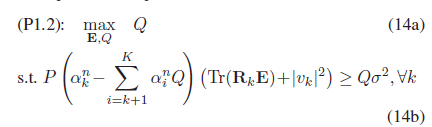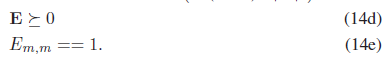# Solve feasibility problem

I want to solve SDP based optimization problem using feasibility method. The problem is formulated asMy code is as follows:

while ( qmax - qmin > tol)

``````q = (qmax+qmin)/2;
``````

cvx_begin
variable QQ(N+1, N+1) hermitian semidefinite;
% feasibility problem
subject to
diag(QQ) == ones(N+1,1);
for j = 1:J
p_uni* (trace(R4(:,:,j)QQ) + (abs(Hd(:,j)))^2) >= p_uniq3((trace(R4(:,:,j)QQ) + (abs(Hd(:,j)))^2))+ qsigma2;
end
cvx_end

% bisection
if strfind(cvx_status,‘Solved’) % feasible
fprintf(1,'Problem is feasible ',q);
qmin = q;
else % not feasible
fprintf(1,‘Problem is not feasible’,q);
qmax = q;
end

end
[Uq, Dq,~] = svd(QQ);

A_Opt = 0;
for iter = 1 : 1
Rq = sqrt(0.5)(randn(N+1, 1) + 1irandn(N+1, 1));
theta = Uq * sqrt(Dq) * Rq ;
theta = theta./(theta(N+1));
theta = theta(1:N)./(abs(theta(1:N)));
A_ite = norm( G * Dh * theta , ‘fro’) ;
if A_ite > A_Opt
A_Opt = A_ite;
theta_opt = theta ;
end
end

Theta_opt = diag(theta_opt);
% total channel with random phase shifts
ht_opt = Hd+h_aiTheta_opth_ui;
abs_h1_opt = sum(abs(ht_opt).^2);

where QQ is the variable E in the picture.
At the end it shows, that the problem is solved, but there is no improvement in the final value abs_h1_opt.
Can anyone help me in this problem? I have been confused for several days and I would appreciate it if anyone can help.

1 Like

What is `abs_h1_optt` and why should it show any improvement?

You have implemented the bisection method for quasi-convex optimization described in section 4,2,5 of https://web.stanford.edu/boyd/cvxbook/, modified to do maximization instead of minimization. This method is to find the optimal (maximum) value of the objective `Q`. So whatever `abs_h1_optt` is, why should it show improvement?

Sorry for the incomplete question.
‘abs_h1_optt’ is the optimized channel gain, which is dependent on optimized phases ‘theta’, obtained from the optimized ‘QQ’. Thus, optimized phase ‘theta’ should improve channel gain ‘abs_h1_optt’, than the case when ‘theta’ is a random vector.

The bisection method is intended to maximize Q subject to the constraints in 14b,c,d. If it did, then it did its job. Whether that problem’s solution results in things dependent on it having the correct or desired properties, pertains to the suitability of the model and modeling approach for whatever real-world phenomenon you are trying to model. That is perhaps an electrical engineering question, not a CVX question. Maybe you should re-read whatever paper or book you got this from in order to better understand the model’s purpose, intended use, and limitations.